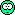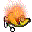The online racing simulator
Is ingame G-meter incorrect?
(4 posts, started )
Is ingame G-meter incorrect?
Hello all.
I found a strange thing. Ingame G-meter shows pisitive longitudinal acceletion when body-speed reducedYou can see it on this video between 11 and 13 seconds

It looks strange.
This video shows difference between rotation of acceleration vector by heading and velocity angle.

gg_x_h = x * cos_h + y * sin_h;
gg_y_h = -x * sin_h + y * cos_h;
Right GG: velocity vector
vel_vec = Math.Atan2(-LFS.OSMain.Vel.X, LFS.OSMain.Vel.Y);
cos = Math.Cos(vel_vec);
sin = Math.Sin(vel_vec);
gg_x = x * cos + y * sin;
gg_y = -x * sin + y * cos;

So. is it bug or feature?
*Drives FZ5*#3
The acceleration meter only gives you values based on the car's heading. It doesn't take into account your velocity vector.

At 0:08 in the first video:

velocity vector: 12 degrees right of car's heading
longitudinal acceleration: 0.20 g
lateral acceleration: 1.25 g
total acceleration: 1.27 g
acceleration vector: 80.9 degrees left of car's heading
acceleration vector: 92.9 degrees left of velocity vector

So the acceleration relative to the velocity vector does have a rearward component, that's why you're slowing down, despite the g meter showing a forward acceleration.
Attached images
#4
S1 track and S2 car on demo license, hmmm

Is ingame G-meter incorrect?
(4 posts, started )

© 2002-2023 Live for Speed - Scawen Roberts, Eric Bailey, Victor van Vlaardingen
All timestamps on this website are displayed in your local timezone
FGED GREDG RDFGDR GSFDG